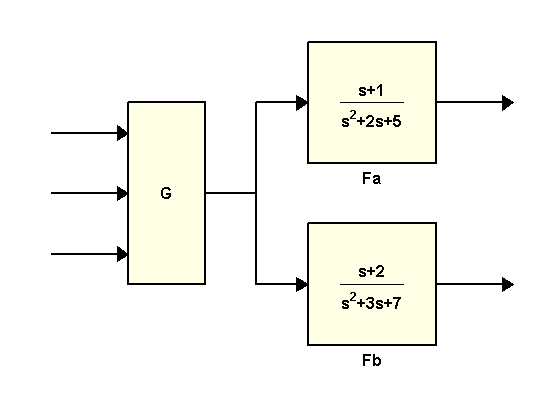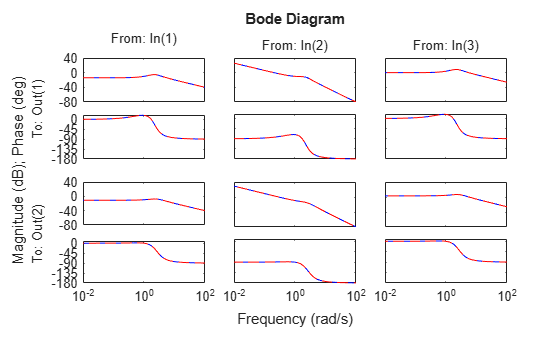# Preventing State Duplication in System Interconnections

This example shows guidelines for building minimum-order models of LTI system interconnections.

### Model Interconnections

You can connect LTI models using the operators `+`, `*`, `[,]`, `[;]` and the commands `series`, `parallel`, `feedback`, and `lft`. To prevent duplication of some of the dynamics and ensure that the resulting model has minimal order, it is important that you follow some simple rules:

• Convert all models to the state-space representation before connecting them

• Respect the block diagram structure

• Avoid closed-form expressions and transfer function algebra.

As an illustration, this example compares two ways to compute a state-space model for the following block diagramwhere

```G = [1 , tf(1,[1 0]) , 5]; Fa = tf([1 1] , [1 2 5]); Fb = tf([1 2] , [1 3 7]);```

### Recommended Method

The best way to connect these three blocks is to convert them to state space and treat the block diagram as a series connection of `G` with `[Fa;Fb]`:

`H1 = [ss(Fa) ; Fb] * G;`

To find the order of `H1`, type

`order(H1)`
```ans = 5 ```

The order 5 is minimal. Note that because SS has higher precedence than TF, it is enough to convert one of the blocks to state-space (the remaining conversions take place automatically).

### Order-Inflating Method

Observe that the overall transfer function is

`$H\left(s\right)=\left(\begin{array}{c}{F}_{a}\left(s\right)G\left(s\right)\\ {F}_{b}\left(s\right)G\left(s\right)\end{array}\right)$`

Therefore, you can also connect the three blocks and compute `H` by typing

`H2 = ss([Fa * G ; Fb * G]);`

Verify that the frequency responses of `H1` and `H2` match:

`bode(H1,'b',H2,'r--')`While `H2` is a valid model, its order is 14, almost three times higher than that of `H1`:

`order(H2)`
```ans = 14 ```

`H2` has higher order because:

• `G` appears twice in this expression

• The dynamics of `Fa` and `Fb` get replicated three time when evaluating `Fa*G` and `Fb*G`

• The state-space conversion is performed on a 2x3 MIMO transfer matrix with four entries of order 2 and two entries of order 3, yielding a total order of 14.

Using a closed-form expression for the overall transfer function is a bad idea in general as it will typically inflate the order and introduce lots of cancelling pole/zero dynamics.

### Conclusion

When connecting LTI models, avoid introducing duplicate dynamics by staying away from closed-form expressions, working with the state-space representation, and breaking block diagrams down to elementary series, parallel, and feedback connections. When in doubt, use the function `connect` which automatically converts all models to state space and is guaranteed to produce minimal realizations of block diagrams.